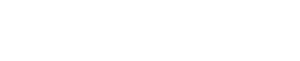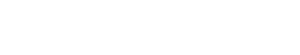# Lambda Functions: Powerful Tools for Data Transformation and Filtering in Real-Time Software Development

In software development, a lambda function, also known as an anonymous function or a function literal, is a small piece of code that can be defined inline without a specific name. Lambda functions are commonly used in functional programming languages and are increasingly popular in many modern programming languages, including Python, JavaScript, and Java.

The term “lambda” comes from lambda calculus, a mathematical system developed in the 1930s, which is the foundation of functional programming. Lambda functions allow you to treat functions as first-class citizens, meaning they can be assigned to variables, passed as arguments to other functions, and returned as values from other functions.

## Lambda functions are particularly useful in real-time software development for several reasons:

1. Concise and Readable Code: Lambda functions enable you to define small, focused pieces of code inline, making your code more concise and easier to read. They are often used for short, one-off tasks that don’t require a separate named function.
2. Callback Functions: In event-driven programming or asynchronous operations, lambda functions can be used as callback functions. For example, when you want to execute a specific action after an event occurs or an asynchronous operation completes, you can pass a lambda function as a callback to be invoked at the appropriate time.
3. Higher-Order Functions: Lambda functions are commonly used in conjunction with higher-order functions, which are functions that accept other functions as arguments or return functions as results. Higher-order functions allow you to abstract and encapsulate common patterns of behavior. Lambda functions provide a convenient way to define these functions inline.
4. Data Transformations: Lambda functions are handy when you need to transform or filter data. For instance, in functional programming, you can use lambda functions with higher-order functions like `map`, `filter`, and `reduce` to perform operations on lists or collections of data.
5. Anonymous Event Handlers: In graphical user interfaces (GUI) or web development, lambda functions can be used as anonymous event handlers. Instead of defining a separate named function to handle an event, you can define a lambda function inline as the event handler, reducing the need for creating multiple functions.

Overall, lambda functions provide a flexible and concise way to work with functions in real-time software development, promoting code reusability, readability, and improved development efficiency.

### Let’s dive deeper into the use of lambda functions with the `map`, `filter`, and `reduce` functions.

1. `map` function:

The `map` function applies a given function to each item in an iterable (e.g., a list) and returns an iterator with the results. It python training in Pune allows you to transform each element in a collection based on a specific operation defined by a lambda function.

The general syntax of `map` is:

map(function, iterable)

Here, `function` is the lambda function that defines the transformation logic, and `iterable` is the collection of elements to be transformed.

Example:In this example, the lambda function `lambda x: x**2` squares each number in the `numbers` list, and the `map` function applies this function to each element, returning a new list of `squared_numbers` with the squared values.

1. `filter` function:

The `filter` function applies a given function to each item in an iterable and returns an iterator with the items for which the function returns `True`. It allows you to selectively filter elements from a collection based on a specific condition defined by a lambda function.

The general syntax of `filter` is:

filter(function, iterable)

Here, `function` is the lambda function that defines the filtering condition, and `iterable` is the collection of elements to be filtered.

Example:In this example, the lambda function `lambda x: x % 2 == 0` checks if a number `x` is even. The `filter` function applies this function to each element in the `numbers` list and returns a new list `even_numbers` with only the even values.

1. `reduce` function:

The `reduce` function, available in the `functools` module in Python, performs a cumulative computation on an iterable by applying a function to pairs of elements from left to right, reducing them to a single value. It is useful when you need to perform operations like summation, multiplication, or any other cumulative computation.

To use `reduce`, you need to import it:

from functools import reduce

The general syntax of `reduce` is:

reduce(function, iterable[, initializer])

Here, `function` is the lambda function that defines the reduction operation, `iterable` is the collection of elements to be reduced, and `initializer` (optional) is the initial value of the reduction.

Example:In this example, the lambda function `lambda x, y: x + y` adds two numbers `x` and `y`. The `reduce` function applies this function cumulatively to the elements of the `numbers` list from left to right, resulting in the sum of all the numbers.

It’s worth mentioning that starting from Python 3, the `reduce` function has been moved to the `functions` module. Therefore, you need to import it explicitly.

These examples illustrate how lambda functions can be used with `map`, `filter`, and `reduce` to perform various operations on collections of data. The flexibility and conciseness of lambda functions make these functional programming techniques powerful tools for data transformation, filtering, and cumulative computations.